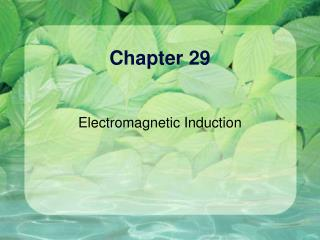DownloadDownload PresentationChapter 29

# Chapter 29

Télécharger la présentation## Chapter 29

- - - - - - - - - - - - - - - - - - - - - - - - - - - E N D - - - - - - - - - - - - - - - - - - - - - - - - - - -
##### Presentation Transcript

1. Chapter 29 Electromagnetic Induction

2. Induced current You mean you can generate electricity this way??!

3. For my next magic act… Note: No moving parts

4. Summary

5. Faraday’s Law of Induction An emf is induced when the number of magnetic field lines that pass through the loop changes

6. Magnetic Flux Similar to electric flux

7. Magnetic Flux

8. Faraday’s Law (restated) The minus sign will be explained later

9. What if you have a coil?

10. EMF induced in a solenoid A=1m2, N=2000 turns An external magnetic field of B = 1mT is removed suddenly in 1s. What is the emf generated?

11. Solution A=1m2, N=2000 turns An external magnetic field of B = 1mT is removed suddenly in 1s. What is the emf generated?

12. Lenz’s Law An induced current has a direction such that the B field due to the current opposes the change in the magnetic flux

13. Lenz’ Law – Example 1 • When the magnet is moved toward the stationary loop, a current is induced as shown in a • This induced current produces its own magnetic field that is directed as shown in b to counteract the increasing external flux

14. The Logic • Bext:  • Bext: increasing • BI:  (to oppose the increase) • I: counterclockwise (view from left)

15. Lenz’ Law – Example 2 • When the magnet is moved away the stationary loop, a current is induced as shown in c • This induced current produces its own magnetic field that is directed as shown in d to counteract the decreasing external flux

16. The Logic • Bext:  • Bext: decreasing • BI:  (to slow down the decrease) • I: clockwise (view from left)

17. Summary

18. Direction of current What is the direction of current in B when the switch S is closed? I

19. Do it yourself! Which way do the currents flow?

20. What is the current? Resistance: R

21. What is the force? Resistance: R

22. Displacement Current There is something wrong with Ampere’s Law Depending on the surface, Iencl could be either zero or non-zero. Inside the capacitor there is no conduction current.

23. Displacement Current We need to account for the E field in Ampere’s Law.

24. Does it work?

25. Displacement current density

26. Example What is the B field at point a given IC?

27. Ampere-Maxwell law

28. Isolated rod vs closed circuit

29. Eddy Currents Eddy currents want to stop whatever you are doing!

30. Which one falls faster?

31. Movie

33. Magnetic materials • Diamagnetism • Paramagnetism • Ferromagnetism

34. Diamagnetism No net magnetic dipole for each atom when B=0. When magnetic field is switched on, an induced magnetic dipole points in the opposite direction to B due to Lenz’s Law, this causes the object to be repelled. Copper, lead, NaCl, water, superconductor

35. Paramagnetism • Each atom already has a permanent dipole moment. • This dipole will align with external B field. • Forces points from weak field to strong (attraction). Oxygen, aluminum, chromium, sodium

36. Movie Liquid Oxygen

37. Ferromagnetism • Each atom has a net magnetic dipole. • Atoms arrange themselves into domains. • External fields can affect the alignment of the domains. • Heat can destroy the domains. • Magnets are made this way. B Field Insert Picture Iron, Permalloy

38. Details

39. Picture

40. Applications of Faraday’s Law • Power plants • Flashlight with no battery • Toothbrush? • Transformers (a.c. versus d.c.)

41. The wonders of magnetic field

42. View from afar

43. Big magnetic field